# Equilibrium Valuation Model: A Regression-Based Fundamental Equity Valuation Model

|
Includes: AAP, AAPL, BBBY, BLK, CSCO, GWW, MU, ORCL, RL, ROST, WMT
by: Boris Wang
Summary

This article proposes a systematic regression-based fundamental equity valuation model that can potentially be applied in areas such as quantitative finance and machine learning.

The model aims to integrate the various fundamental factors of equity valuation into a unified regression function capable of predicting the value of individual stocks in an equilibrium market state.

A sample regression model built to test the theory is able to predict the historical stock prices of companies in the S&P 500 with a MAPE of 34.94%.

This article (original research paper) proposes a systematic regression-based fundamental equity valuation model that can potentially be applied in areas such as quantitative finance and machine learning. The proposed model aims to methodically integrate the various fundamental factors of equity valuation, such as free cash flow, EBITDA, revenue growth, operating margin, capital expenditures, etc., into a unified regression function capable of predicting the value of individual stocks in an equilibrium market state.

This article will first explain the theory underlying the proposed model; beginning from the fundamental theory of asset valuation, to its application in equity valuation, then to the derivation of the proposed model. Next, methodologies to test the proposed model will be described. And finally, results and outputs of the test model will be discussed along with suggestions for future research.

### Theory

Assumptions

The underlying theory of the proposed model assumes the following conditions:

• Competitive market
• Minimal transaction costs
• No restrictions on short-selling or borrowing
• Investors seek to maximize economic returns
• Investors are rational and risk-neutral
• All assets are perfectly liquid and divisible
• All information are available to all investors at the same time

Fundamentals of Valuation

The value of an asset is determined by three fundamental factors, income, risk, and risk-free rate.

Income is the basis of value and is reflected by the yield, which measures the amount of income as a percentage of the asset price: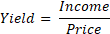Conversely, yield is also a measure of valuation, as the reciprocal of yield is the price-to-earnings ratio, which reflects how much a certain amount of income is valued: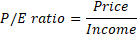In the condition of equal risk and risk-free rate, the law of one price asserts that every asset must have the same yield. Suppose there are only two assets in the market, a \$50 stock which generates \$5 of free cash flow per year, and a \$100 bond which pays \$20 of interest per year: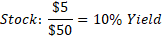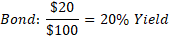If the presupposed condition holds, then investors will seek the higher yield by selling the stock and buying the bond. This arbitrage will drive the price of the stock down and the price of the bond up until the yields of both assets converge to an equilibrium rate of 15%, at which then investors would be indifferent to buying either the stock or the bond: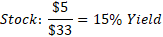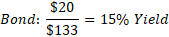Variations in yields exist due to risk, or the uncertainty in realizing the income. Alternatively, risk can be defined as the existence of stochastic states in which the realized income does not equate to the stated income.1 Income adjusted for risk is reflected by the expected return: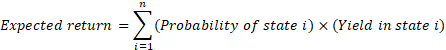In the condition of equal risk-free rate, the law of one price asserts that every asset must have the same expected return.2 Suppose there are only two assets in the market, a risk-free bond that is guaranteed to pay interest every year and a risky bond that has a 50% chance of not paying interest each year, both priced at 20% yield: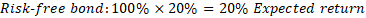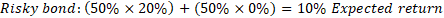If the presupposed condition holds, then investors will seek the higher expected return by selling the risky bond and buying the risk-free bond. This arbitrage will drive the yield of the risky bond up and the yield of the risk-free bond down until the expected returns of both bonds converge to an equilibrium rate of 15%, at which then investors would be indifferent to buying either bonds: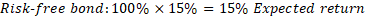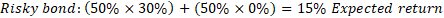An implication of the law of one price is the existence of an equilibrium expected return by which all assets are valued. Alternatively, this equilibrium expected return can be viewed as the risk-free rate.

The risk-free rate is the yield that a hypothetical risk-free asset must have; this is derived based on the assertion that the yield of a risk-free asset must equate to its expected return (due to the certainty of its income), and that its expected return must equate to the equilibrium expected return (due to the law of one price):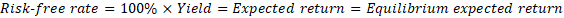The law of one price asserts that every asset must have an expected return equal to the risk-free rate. Suppose a market has a risk-free rate of 10%; if a stock with an expected return of 20% is added to the market, then investors will seek the higher expected return by selling other assets in the market (which must all have an expected return of 10%) and buying the stock. This arbitrage will drive the expected return of the stock down until it converges to the risk-free rate of 10%, at which then investors would be indifferent to buying any asset in the market (assuming the stock does not affect the equilibrium expected return of the market).

In summary, the value of an asset is a function of the income it generates, the uncertainty of realizing the income, and the risk-free rate of the market; this can be stated as the following: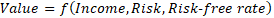Equity Valuation

The three fundamental factors of valuation are reflected in equity valuation through the discounted cash flow (DCF) model, which is used to calculate a stock's present value.3

The income factor in equity valuation is represented by free cash flow (FCF), which reflects the amount of cash income generated by a company's operations that is freely distributable to the company's shareholders after deducting for expenditures required to maintain and to expand operations on a going concern basis.4

The risk factor in equity valuation is represented by the expected FCF growth rate, which is adjusted to FCF to derive the expected FCF. An intuitive way to conceptualize equity risk is to view the stock as a constant perpetuity.5 A constant perpetuity can be considered risk-free when there is no uncertainty or potential change to its constant coupon payments. Quantitatively, this risk-free perpetuity can be described as having an expected coupon growth rate of 0%.6

Assuming if the perpetuity has a 50% probability of not paying its coupon next year, then it would no longer be considered risk-free as its constant coupon payment is now subject to uncertainty and has the potential to change. Quantitatively, this risky perpetuity's expected coupon growth rate for the next year can be expressed as -50%, which reflects the expected change in the value of next year's coupon: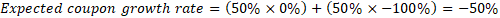For assets with constant income, risk-free can be understood as the absence of potential change and can be expressed by a 0% expected growth rate; and risk can be understood as the potential for change and can be expressed by a non-zero expected growth rate.7 For assets with variable income, such as equities, the same conception of risk can be applied by viewing the current income as the constant income.

For example, a stock, with its current FCF being the "constant coupon payment", can also be considered "risk-free" if it has an expected FCF growth rate of 0%. And if there is a probability that FCF will decline in the future (relative to the current FCF), then this would represent a potential downward change (downside risk) in FCF and it can be expressed as a negative expected FCF growth rate. When inputted into the DCF model, the negative growth rate decreases the valuation of the stock relative to its current FCF, which results in a higher FCF yield and a lower multiple. This is consistent with the observation that higher risk correlates with higher yield.

And if there is a probability that FCF will increase in the future, then this would represent a potential upward change (upward risk) in FCF and it can be expressed as a positive expected FCF growth rate. When inputted into the DCF model, the positive growth rate increases the valuation of the stock relative to its current FCF, which results in a lower FCF yield and a higher multiple. This is consistent with the observation that lower risk correlates with lower yield.8

The risk-free rate factor in equity valuation is represented by the discount rate, which is used to discount a stock's expected FCF to its present value. By discounting expected FCF with the risk-free rate - commonly represented by the government bond yield, the expected return of the stock is thus equated to the equilibrium expected return of the market.

Finally, the value of a stock as derived by the DCF model can be expressed as the following: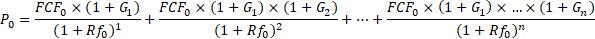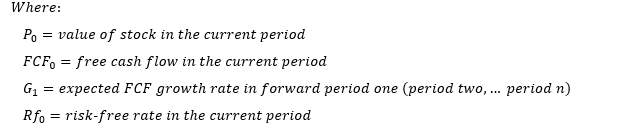Equilibrium Valuation Model

Based on the DCF model, it can be stated that the current value of a stock (P0) is a function of its current FCF (FCF0), its future expected FCF growth rates (G1, G2, …Gn), and the current risk-free rate (Rf0). Assuming if G1, G2, …Gn can be expressed as a function representing the total time-adjusted expected FCF growth potential of a stock at the current period (TG0), then it can be stated that P0 is a function of the following three factors: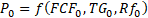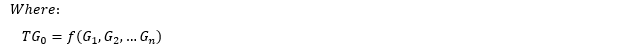Moreover, assuming the law of one price holds, then it should be possible to derive such a valuation function capable of determining P0 in a market equilibrium state based on the three valuation factors. This concept is illustrated through the following example.

Consider a hypothetical perfect market which is composed of the following stocks: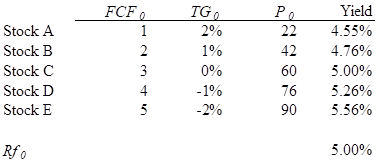Based on these data, the relationship between P0 and the three valuation factors can be derived as the following:9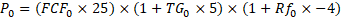This multivariable function is essentially the market's equilibrium valuation function, as it defines how the market values each of the three valuation factors and how these factors interact in an equilibrium state. For example, if Stock F, which has a FCF0 of \$6 and a TG0 of 1%, is added to the market, then its P0 as determined by the equilibrium valuation function would be \$126 (4.76% yield):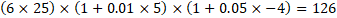If Stock F is instead priced at \$120 (5.00% yield), then it would represent a mispricing and would allow investors to earn arbitrage profit (\$6) by buying one Stock F (\$120), splitting it into three stocks (each with a FCF0 of \$2 and a TG0 of 1%), and selling them as Stock B (\$42 each or \$126 in total). This arbitrage will result in P0 of Stock F converging to \$126 to reflect the equilibrium valuation of the market (assuming the arbitrage does not affect the valuation of Stock B).

And if Stock F is priced at \$130 (4.62% yield), then investors can earn arbitrage profit (\$4) by buying three Stock B (\$42 each or \$126 in total), combining them into a single stock (with a FCF0 of \$6 and a TG0 of 1%), and selling it as Stock F (\$130). Again, this arbitrage will result in P0 of Stock F converging to \$126 to reflect the equilibrium valuation of the market.

There are two specific assumptions underlying the derivation of this equilibrium valuation function. The first assumption is TG0, a theoretical factor representing the totality of a stock's time-adjusted expected FCF growth potential as of the current period. Quantitatively, a stock's TG0 can be defined as the input variable which, together with the stock's FCF0 and Rf0, equates the output of the equilibrium valuation function, P0, to the stock's hypothetical fair value.

In application, TG0 can be approximated by calculating a stock's total expected FCF growth rate for the forward n periods.10 Generally, stocks are valued using the DCF model on a 10-year forward-looking time horizon before a generic terminal value is imposed; this means that the company-specific portion of a stock's expected FCF growth potential is derived from only 10 years of growth estimates. Therefore, the total expected FCF growth rate for the forward 10 years should serve as a relatively accurate proxy for TG0; this can be expressed as the following:11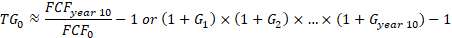The second assumption required for the derivation of the equilibrium valuation function is the law of one price. Note that in the example given, the equilibrium valuation function is a perfect predictor of stock price because the market it is derived from is perfect and there is no mispricing among the existing stocks (due to the law of one price). In application, however, the derived function may not be perfect due to possible inefficiencies in the market, and the degree of its prediction accuracy will likely correlate with the degree of the market's efficiency.

Therefore, in order to derive a sufficiently predictive equilibrium valuation function, it is required that the stock market be mostly efficient. Though individual stocks may be mispriced at certain periods of time, the deviation between current price and fair value should be averaged out when applied to the market as a whole and over time. Therefore, if on average the stock market is accurately valued, then the equilibrium valuation function derived from it should also be accurately calibrated.

Assuming that TG0 can be approximated using forward FCF growth estimates and that the market is mostly efficient, then theoretically it should be possible to derive from the equity market a sufficiently predictive equilibrium valuation function capable of predicting the value of individual stocks.

Factor Modularization

The "equilibrium valuation model" in its basic theoretical form is defined as the following: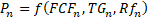In application, however, the model in its basic form may be insufficient in reflecting the underlying fundamental aspects of each valuation factor. For example, suppose there are two stocks with the following FCF components:12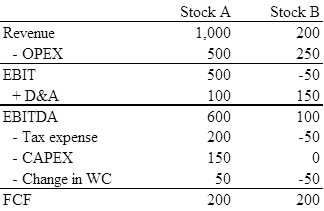Though these two stocks have the same FCF, it is apparent from a fundamental analysis perspective that Stock A has the "higher quality" FCF. First, Stock A is generating positive operating income, whereas Stock B is losing money on its operations. Second, Stock A is investing in its future business as can be seen from its positive net capital expenditures and working capital spending, whereas Stock B is not spending on capital expenditures and is expending its current working capital.13 However, by having the same FCF, the model would be unable to identify these fundamental differences between Stock A and Stock B.

Theoretically, the discrepancy between a stock's FCF and its underlying "quality" should be reflected through TGn. For example, if Stock A is generating positive operating income and is investing in its future business, then its TG0 should be higher than Stock B, which is losing money on operations and is not investing in its business. Therefore, even though both stocks currently generate the same FCF, the model will correctly produce a higher valuation for Stock A due to its higher TG0 (assuming positive correlation with value). However, because TGn is approximated using only 10 years of expected FCF growth rates, the possibility of this fundamental discrepancy lasting for longer periods can render TGn ineffective in correcting any distortions created by a "low quality" FCFn.

This shortcoming of the model can be resolved through factor modularization, a process by which each basic factor is divided and represented by incrementally more precise sub-factors. For example, FCFn can be represented by the following components:14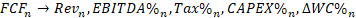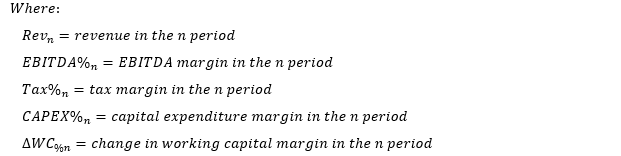In this breakdown of FCF, Revn represents the gross cash inflow received from operations; EBITDA%n represents the percentage of Revn retained as operating income; Tax%n, CAPEX%n, and ∆WC%n represent the percentage of Revn paid out as tax expense, capital expenditures, and working capital. By representing FCFn with its components, it allows the model to identify the relative importance (coefficient) of each component and as a result provide more precise representations of the fundamental aspects underlying a stock's FCF.

To correspond with the FCFn components, TGn can be divided into the following: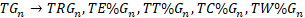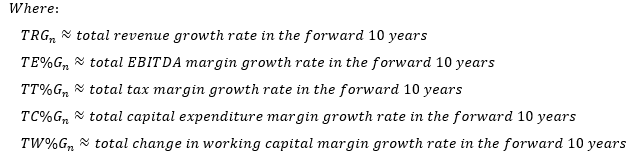Using these components to represent TGn not only more precisely reflects the growth potential of a stock, but also more closely aligns with how the market views and estimates growth. For example, when analysts estimate the forward growth rate of a stock, they typically do so by separately forecasting the company's revenue growth rate, the change in EBITDA margin, and changes in other expense margins.

Factor modularization can be applied to Rfn by representing it with multiple durations of the government bond yield such as the following: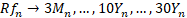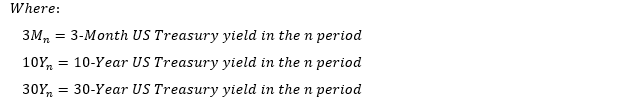This allows for a more comprehensive representation of Rfn by enabling the model to identify the coefficient of each duration and measure how different durations affect stock valuation.

It is also possible to apply factor modularization to the model's output, Pn, by dividing it into components and using the most precise component as its representation. For example, the measure of stock value, market capitalization (or stock price on a per share basis), can be calculated as the following: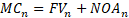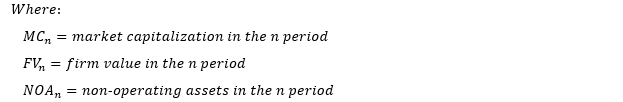Firm value represents the value of a company's business operations, whereas non-operating assets represent items not directly related to operations such as cash, investments, debt, preferred securities, non-controlling interest, etc.15 In this case, firm value can be used to represent Pn as it is the more direct and precise measure of a stock's underlying business value: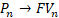With factor modularization, the Equilibrium Valuation Model can be stated in the following expanded form: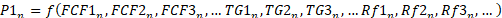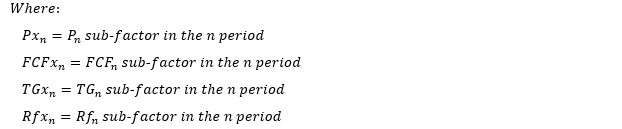Theoretically, the factor modularization process can be repeated indefinitely to yield incrementally more precise representations of the basic factors. For example, EBITDA%n can be further divided into operating expense margin (OPEX%n) and depreciation and amortization margin (D&A%n); OPEX%n can also be further divided into cost of goods sold margin (COGS%n), sales, general, and administrative margin (SG&A%n), and research and development margin (R&D%n), etc.

In application, however, factor modularization is constrained by the variations in company financial reporting and business models. For example, if sub-factors such as EBITDA%n, CAPEX%n, and ∆WC%n are used to represent FCFn, then this model would be inapplicable to stocks in the financial sector, such as banks and insurance companies, as their operations cannot be cleared defined in terms of EBITDA, capital expenditures, or working capital (see "Valuing Financial Service Firms" by Aswath Damodaran). And if EBITDA%n is further divided into COGS%n, SG&A%n, R&D%n, and D&A%n, then this model would be inapplicable to stocks that do not report cost of goods sold or research and development expenses.

Therefore, the degree of factor modularization is dependent on the trade-off between precision and scope. If the scope is to derive an equilibrium valuation function applicable to the entire market, then more basic factors should be used to accommodate the variety of different stocks in the market; and if the scope is to derive an equilibrium valuation function for a specific sector of the market, then more precise and specific factors can be used to achieve greater accuracy and descriptive capabilities.

### Methodology

Identifying Limitations

To test the Equilibrium Valuation Model in application, a sample regression model is built based on the methodologies described in the subsequent sections. First, it is necessary to identify the major limitations subjected by the test model:

• In theory, TGn and its sub-factors should be approximated using the forward 10 years growth estimates. However, due to the unavailability of these data, TGn and its sub-factors in the test model are instead approximated using historical growth rates based on the most recent period.16
• The Equilibrium Valuation Model as it is currently constructed does not incorporate the effects of debt and financial leverage. Therefore, the test model will not account for these factors.17
• Company financial information used in the test model are obtained from third-party sources and are susceptible to various errors such as misplaced, omitted, and inaccurate numbers.

Specifying Variables

The test model is built using the following variables: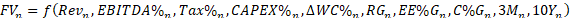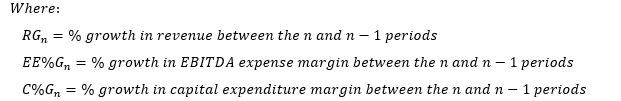The EBITDA calculation of FCF is used (instead of EBIT) as it provides a more accurate representation of a company's operating performance by ignoring non-cash depreciation and amortization expenses.18

To avoid division errors/distortions caused by a zero/negative EBITDA margin denominator, EBITDA margin growth rate in the model is instead represented by the EBITDA expense margin growth rate.19

Tax margin growth rate and change in working capital margin growth rate are excluded from the model as they are generally immaterial and are typically set as a percentage of revenue for going concern companies.

Organizing Data

Data for the test model are obtained from companies in the S&P 500 - widely considered to be the most efficient segment of the US equity market.

In order to construct a comparable and standardized data sample, companies not applicable to the EBITDA approach of calculating FCF and companies in which TGn may not be reliably approximated (using historical growth rates) are excluded from the sample. This includes companies that are significantly impacted by specific macro-economic factors (such as energy and material companies), companies that operate on balance-sheet-centric business models (such as banks, insurance companies, REITs, and certain other financial services companies), and companies that operate on other non-standard business models (such as patent-dependent healthcare companies). After these exclusions, there are in total 283 companies in the sample.20

With fiscal quarters being the basic unit of time, quarterly financial data for each sample company going back to the year 1996 (if available) are inputted into the following Excel company template (Apple (AAPL) will be used as the example hereafter):21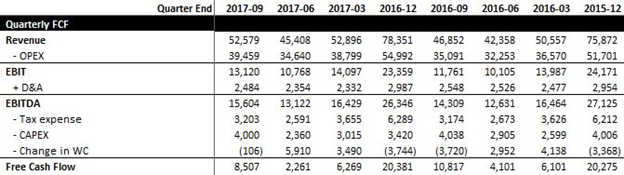Source: Created by author using data from YCharts.com

In order to accurately represent the "true" FCF of each company, every company within the sample is manually screened and adjusted for material one-time items and company-specific operating items such as deferred revenue and capital/finance leases.22 Furthermore, all negative EBITDA in the sample are set to zero as EBITDA becomes uncorrelated with value when negative (companies are only valued based on expectations of positive EBITDA).

To eliminate the effect of cyclicality and to provide an annualized representation of FCF, the quarterly FCF data are then converted to the trailing 12 months (TTM) format to arrive at the TTM FCF data (and Revn):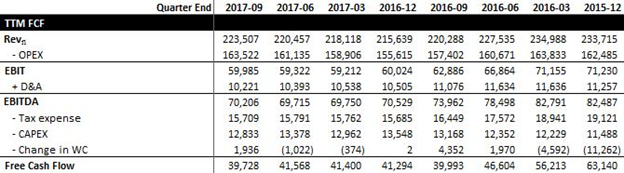Source: Created by author

To correctly align the valuation of a company at a given quarter to the financial information by which the valuation is based on, TTM is calculated as the sum of the n - 1, n - 2, n - 3, and n - 4 quarters (instead of the n, n - 1, n - 2, and n - 3 quarters). This is necessary because in application the valuation of a company at a given quarter is derived based on the company's reported financial information from the preceding quarter.

The other FCF variables are then calculated by dividing the respective TTM FCF data by Revn: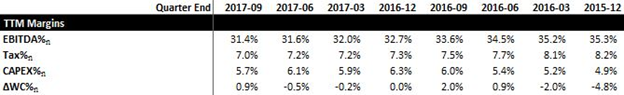Source: Created by author

Next, FCF growth variables are calculated using the FCF variables from the n and n - 1 quarters: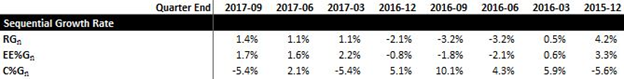Source: Created by author

Then, quarterly average 3-Month and 10-Year US Treasury yields are inputted into each company template in alignment with the company's fiscal quarter:23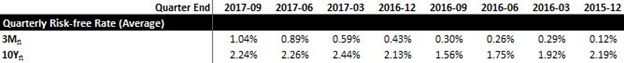Source: Created by author using data from the St. Louis Fed website

Several calculations are made to arrive at a company's firm value. First, quarterly average stock prices (SPn) are inputted into each company template in alignment with the company's fiscal quarter.24 Next, SPn is multiplied by the preceding quarter's weighted-average diluted shares outstanding (WADSn) to derive the quarterly average market capitalization (MCn).25 Lastly, MCn is deducted by the preceding quarter's ending non-operating assets (NOAn) to arrive at the quarterly average firm value (FVn):26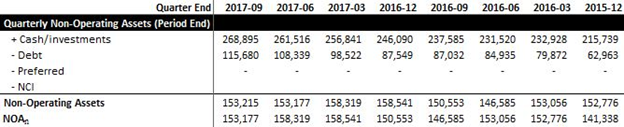Source: Created by author using data from YCharts.com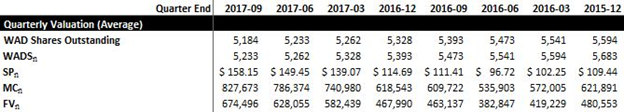Source: Created by author using data from YCharts.com and Yahoo Finance

Finally, quarterly data sets which have incomplete/missing variables are removed (along with all preceding quarters). The remaining complete quarterly data sets from the 283 company templates are then combined and inputted into a single Excel regression model template totaling 20,216 quarterly data sets.

Formulating Function

The next step in building the test model is to formulate the regression function. Because it is not yet known by which relationship the variables and coefficients interact, four different functions are proposed and tested:

Function #1: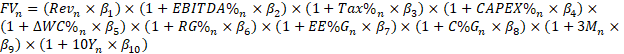Function #2: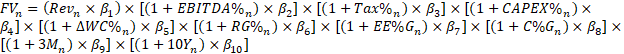Function #3: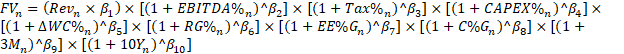Function #4: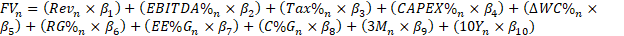After setting up the functions on the regression model template, Excel Solver is used to solve for the optimal coefficients with the goal of minimizing the mean absolute percentage error (MAPE) between the predicted FVn and the actual FVn for all 20,216 quarterly data sets: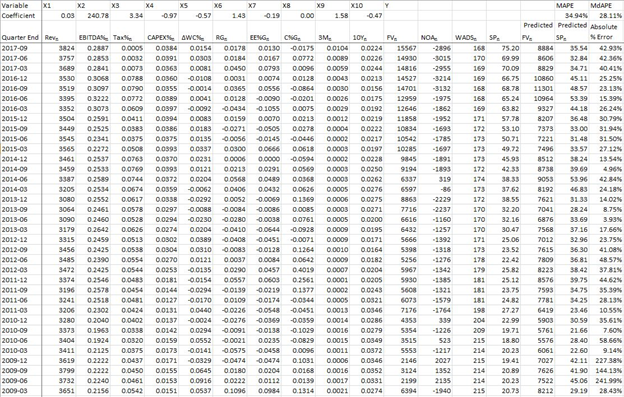Source: Created by author

After the optimization of coefficients, the function which produced the lowest MAPE is considered to be the equilibrium valuation function for the purpose of this test model.

### Result

The four proposed functions yielded the following MAPE after optimization: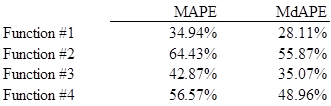Based on these results, Function #1 on average is determined to be more accurate in predicting FVn for the 20,216 quarterly data sets. Therefore, the remainder of this section will focus on the outputs of Function #1, which is derived as the following (coefficients rounded to two decimal places):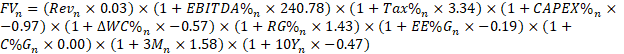The predicted FVn can then be converted into SPn by using the following formula: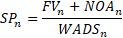Using this test model, it's possible to compare a company's historical SPn with the predicted SPn by generating a cross-sectional graph with time on the x-axis and price on the y-axis. Below is the historical price prediction chart of Apple:27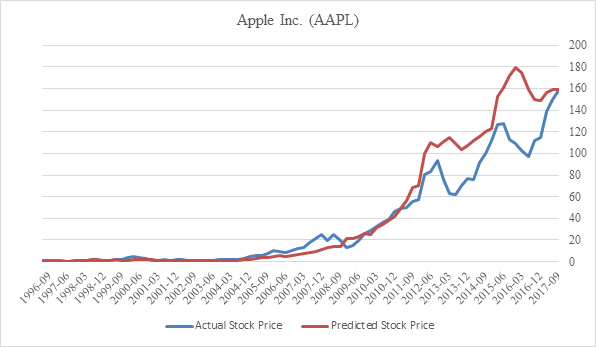Source: Created by author

The test model was able to produce some interesting price predictions which in many cases exhibited leading effects. For example, the historical price predictions for Cisco Systems (CSCO) and Oracle (ORCL) were able to identify the Dot.com Bubble in the early 2000s. Furthermore, in the case of Cisco Systems, the predicted price was able to consistently lead the actual price after 2010, possibly suggesting that the stock was undervalued.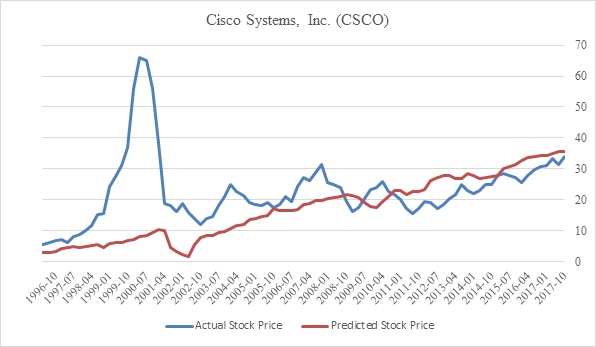Source: Created by author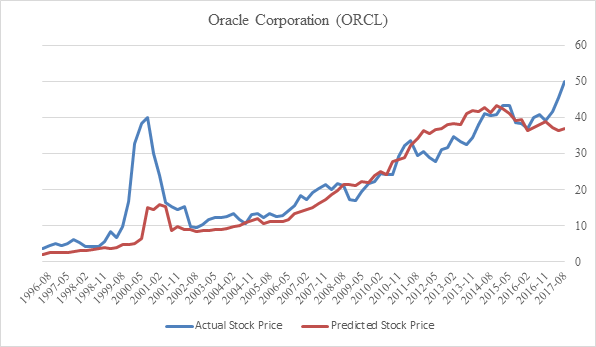Source: Created by author

In the case of Walmart (WMT), the actual price consistently stagnated when it was higher than the predicted price, possibly indicating overvaluation during those years. After the predicted price surpassed the actual price in 2006, the actual price broke out of its stagnation and began rising in accordance with the predicted price.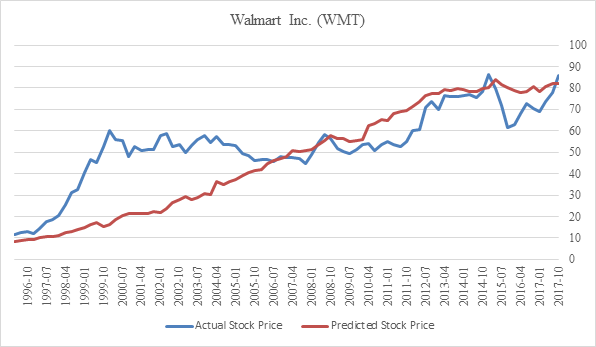Source: Created by author

In the case of Advance Auto Parts (AAP), prior to 2014 the predicted price consistently led the actual price. Though a divergence occurred between the predicted and actual price between 2014 and 2016, the divergence was quickly reduced in 2017. This may be a case where the stock price corrected back to its fair value. Similar cases of divergence and convergence can be observed in stocks such as Ross Stores (ROST), Ralph Lauren (RL), and W. W. Grainger (GWW).Source: Created by author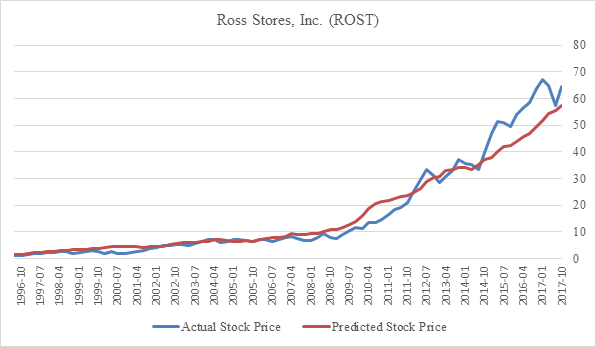Source: Created by author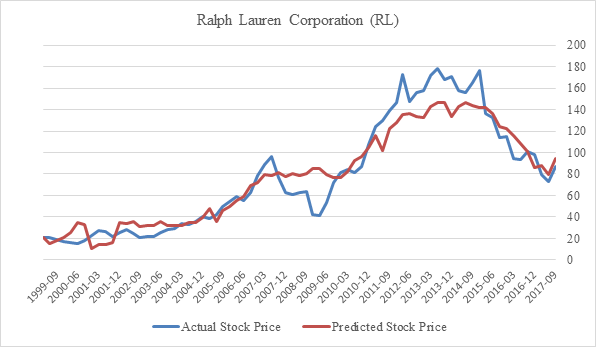Source: Created by author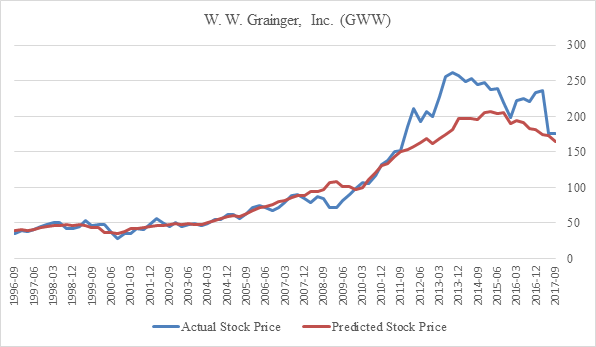Source: Created by author

However, for the majority of the stocks, the predicted price simply mirrored the actual price to different degrees and did not exhibit any noticeable leading effects. The historical price prediction charts of BlackRock (BLK), Bed Bath & Beyond (BBBY), and Micron Technology (MU) are typical examples of the test model's output.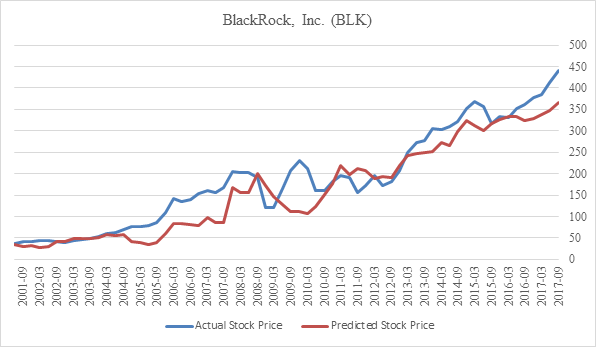Source: Created by author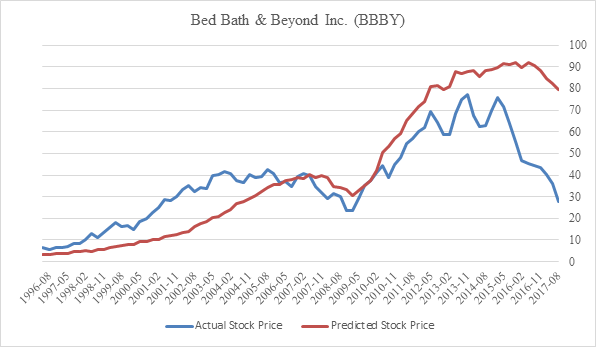Source: Created by author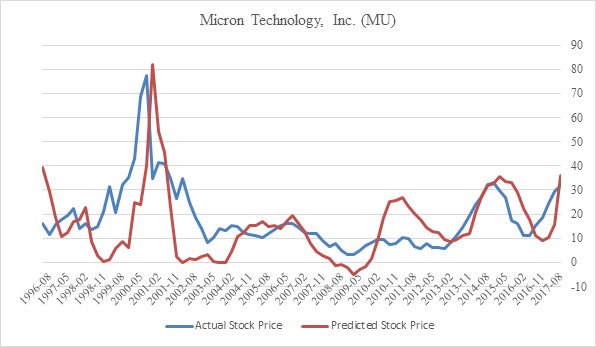Source: Created by author

Based on the overall results, it can be proposed that the test model does indeed possess predictive powers. Though there is a wide range of discrepancies observed, the model is generally able to produce price predictions which approximated the actual prices.

Much of the observed discrepancies between the predicted and actual prices can be attributed to the various limitations subjected by the test model as discussed previously. Therefore, by improving on those limitations, the model's prediction accuracy should increase significantly.

The model's predictive power can also be enhanced by improving the regression function. The test model assumed a highly simplistic representation of the equilibrium valuation function whereby all the variables and coefficients interacted with similar operations. In reality, however, the optimal equilibrium valuation function may interact with much more complex operations.

Finding the optimal function is inherently an iterative task requiring significant computational power. As a result, machine learning techniques such as artificial neural networks may need to be applied in this pursuit (see "Using Artificial Neural Networks to Predict Stock Prices" by Tomasz Kozdraj).

### Conclusion

The implications of the Equilibrium Valuation Model are significant, as further research and development can lead to numerous practical applications such as the identification of mispriced stocks, the calculation of implied risk, and the valuation of private companies. Furthermore, given that the underlying theory of the model is applicable to all assets, it may be possible to apply this model to other asset classes as well.

### Appendix

Disclosure: I/we have no positions in any stocks mentioned, and no plans to initiate any positions within the next 72 hours. I wrote this article myself, and it expresses my own opinions. I am not receiving compensation for it. I have no business relationship with any company whose stock is mentioned in this article.